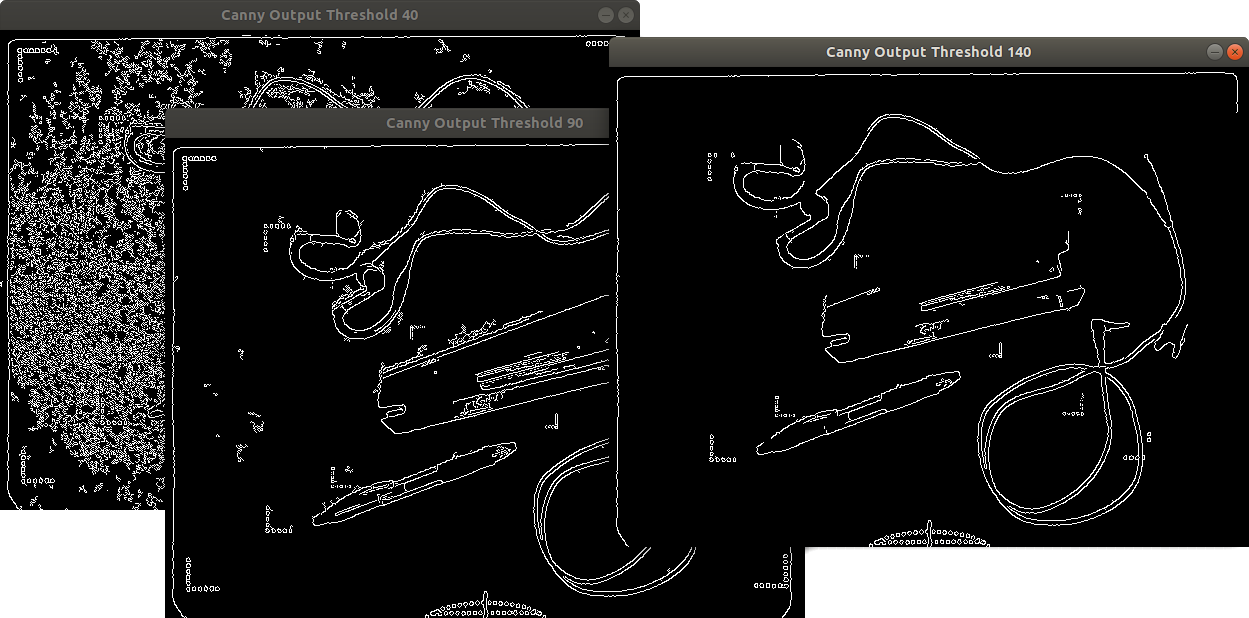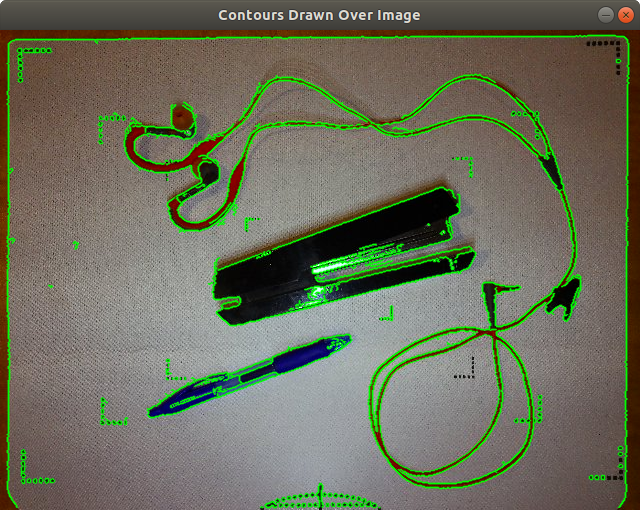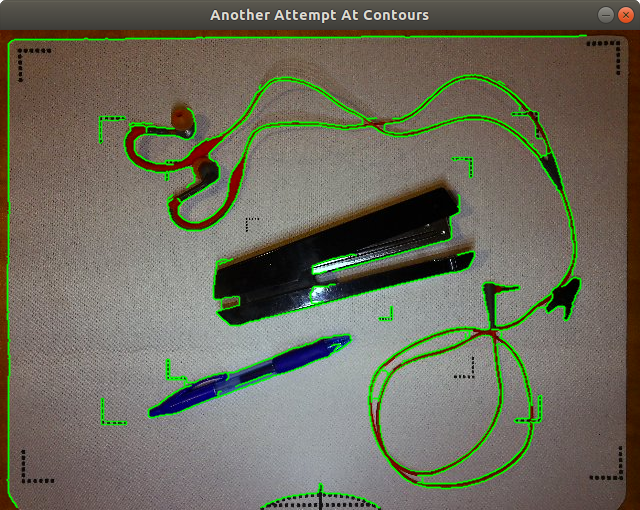../

## Summary

This is the 3rd post in a series on OpenCV. Previously:

## Canny Edge Detection

Canny edge detection takes a colour or grayscale input image and returns a black-and-white image showing all the detected edges. Parameters to cv::canny() determine the level of edge detection. Here is an example with 3 different thresholds:A good starting point for the first threshold is the value returned by cv::threshold() when used with the parameter cv::THRESH_OTSU, as described in the previous post. The second threshold is typically several times larger than the first.

for (double canny_threshold : { 40.0, 90.0, 140.0 } ) { cv::Mat canny_output; cv::Canny(original_image, canny_output, canny_threshold, 3.0 * canny_threshold, 3, true); const std::string name = "Canny Output Threshold " + std::to_string((size_t)canny_threshold); cv::namedWindow(name, cv::WINDOW_AUTOSIZE); cv::imshow(name, canny_output); }

Once edge detection has run, the next step is to find the contours. The input image to cv::findContours() is the binary image from Canny edge detection. The main output is not an image, but a vector of contours, each of which is a vector of points. These points can then be easily drawn to create a new image, or even drawn on top of an existing image. An example will help:

typedef std::vector<cv::Point> Contour; // a single contour is a vector of many points typedef std::vector<Contour> VContours; // many of these are then combined to create a vector of contour points VContours contours; std::vector hierarchy; cv::findContours(canny_output, contours, hierarchy, cv::RETR_EXTERNAL, cv::CHAIN_APPROX_SIMPLE);

If 10 different edges/contours were identified, then contours will contain 10 items. Each of these items is a vector of points. Some contours will be composed of a dozen points, while others may be hundreds or thousands of points.

Many things can be done with contours. You could calculate the area of each individual contour:

for (auto & c : contours) { std::cout << "contour area: " << cv::contourArea(c) << std::endl; }

But possibly more interesting, is to draw the contours on top of the original image so we can visually examine exactly what was detected. One of the parameters to cv::polylines() is a vector of points, each representing the ends of the line to draw. Conveniently, each contour is exactly that -- a vector of points. So starting with the original colour image, we can create a clone (just because I didn't want to make changes to the original image) and draw each contour:

const cv::Scalar green(0, 255, 0); cv::Mat output = original_image.clone(); for (auto & c : contours) { cv::polylines(output, c, true, green, 1, cv::LINE_AA); } cv::namedWindow("Contours Drawn Over Image", cv::WINDOW_AUTOSIZE); cv::imshow("Contours Drawn Over Image", output);

This should give results that look similar to this:False positives can be reduced or eliminated using several different methods:

• You can walk the vector of contours and examine the area or the number of points in each contour to filter out too-small or too-large contours.
• You can tweak the threshold values during Canny edge detection.
• You can blur the image first to reduce the number of small items picked up.
• You can erode and dilate the image to reduce noise.

See the attached source code which uses a combination of Gaussian blur, erosion, and dilation to generate the following boundaries: